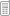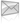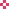+91-9818369374ibworldacademy@gmail.com

# Question

(a)     Find the interval where g¢ (t) > 0.

(4)

(b)     Find the interval where g² (t) > 0 and the interval where g² (t) < 0.

(5)

(c)     Find the value of t where the graph of g (t) has a point of inflexion.

(3)

(d)     Let t* be a value of t for which g¢ (t*) = 0 and g² (t*) < 0. Find t*.

(3)

(e)     Find the point where the normal to the graph of g (t) at the point
(t*, g (t*)) meets the t-axis.

# Answer

(a) Find the interval where g (t) > 0. (4) (b) Find the interval where g (t) > 0 and the interval where g (t) < 0. (5) (c) Find the value of t where the graph of g (t) has a point of inflexion. (3) (d) Let t* be a value of t for which g (t*) = 0 and g (t*) < 0. Find t*. (3) (e) Find the point where the normal to the graph of g (t) at the point (t*, g (t*)) meets the t-axis.

Quick Contact#Ask An Expert

#### Post Your Question Click Here

AssignmentWork Payment (USD)